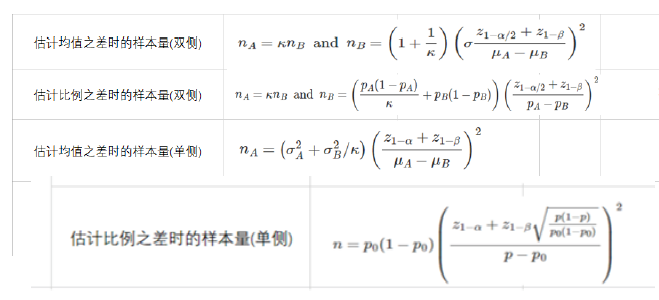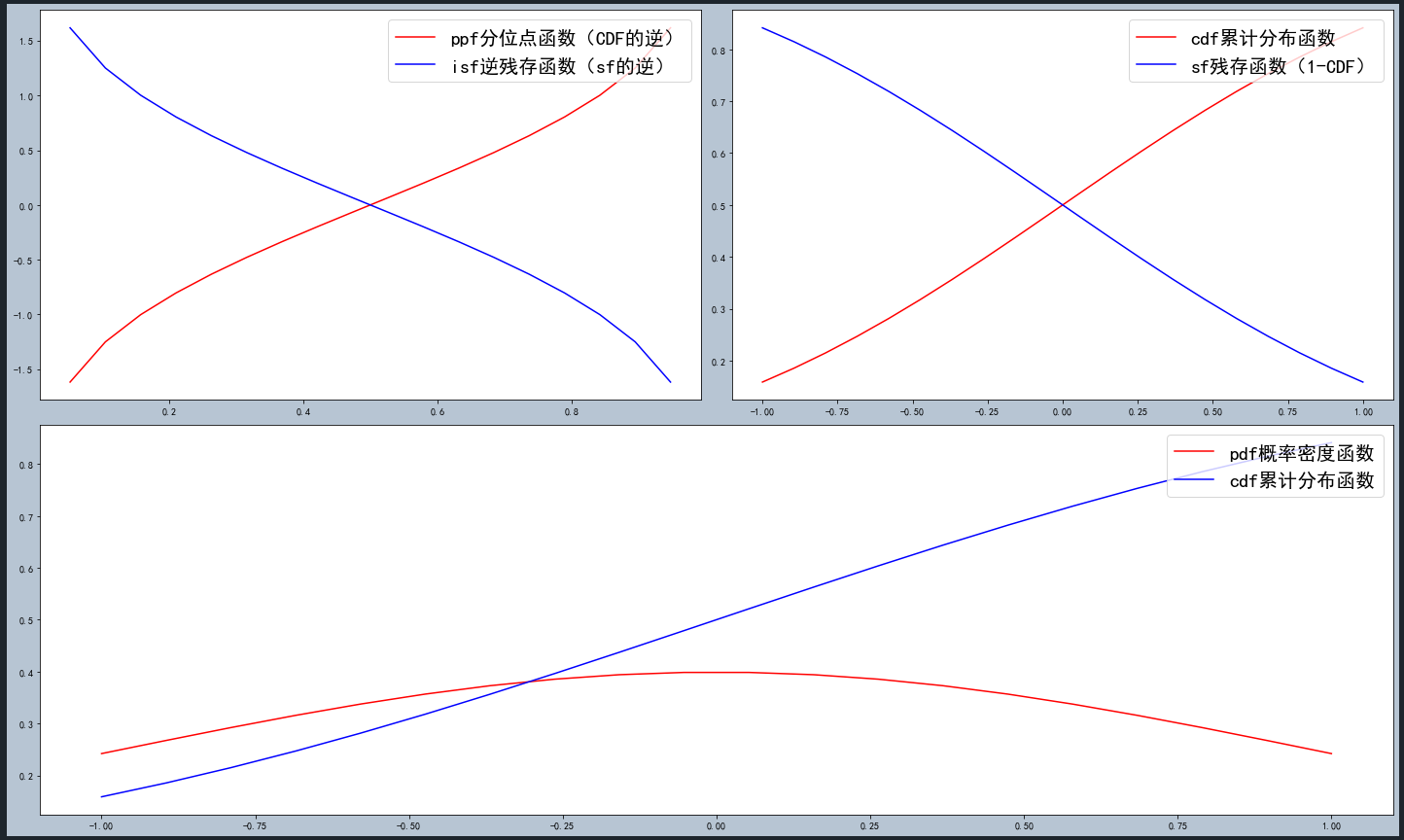• 格式：n=norm(A,p)功能：norm函数可计算几种不同类型的矩阵范数,根据p的不同可得到不同的范数以下是Matlab中help norm 的解释NORMMatrix or vector norm.For matrices...NORM(X) is the largest singular value of X...

格式：n=norm(A,p)

功能：norm函数可计算几种不同类型的矩阵范数,根据p的不同可得到不同的范数

以下是Matlab中help norm 的解释

NORM   Matrix or vector norm.

For matrices...

NORM(X) is the largest singular value of X, max(svd(X)).

NORM(X,2) is the same as NORM(X).

NORM(X,1) is the 1-norm of X, the largest column sum,

= max(sum(abs(X))).

NORM(X,inf) is the infinity norm of X, the largest row sum,

= max(sum(abs(X'))).

NORM(X,'fro') is the Frobenius norm, sqrt(sum(diag(X'*X))).

NORM(X,P) is available for matrix X only if P is 1, 2, inf or 'fro'.

For vectors...

NORM(V,P) = sum(abs(V).^P)^(1/P).

NORM(V) = norm(V,2).

NORM(V,inf) = max(abs(V)).

NORM(V,-inf) = min(abs(V)).

1、如果A为矩阵

返回A的最大奇异值，即max(svd(A))

n=norm(A,p)

根据p的不同，返回不同的值

p

返回值

1

返回A中最大一列和，即max(sum(abs(A)))

2

返回A的最大奇异值，和n=norm(A)用法一样

inf

返回A中最大一行和，即max(sum(abs(A’)))

‘fro’

A和A‘的积的对角线和的平方根，即sqrt(sum(diag(A'*A)))

2、如果A为向量

norm(A,p)

返回向量A的p范数。即返回 sum(abs(A).^p)^(1/p),对任意 1

norm(A)

返回向量A的2范数，即等价于norm(A,2)。

norm(A,inf)

返回max(abs(A))

norm(A,-inf)

返回min(abs(A))

展开全文• 官方文档：...函数norm(input, p='fro', dim=None, keepdim=False, out=None, dtype=None) Returns the matrix norm or vector norm of a given tensor. 参数: input (Tensor) – 输入张量 p (.

官方文档：https://pytorch.org/docs/stable/generated/torch.norm.html?highlight=norm#torch.norm

函数：

norm(input, p='fro', dim=None, keepdim=False, out=None, dtype=None)
Returns the matrix norm or vector norm of a given tensor.

参数:

• input (Tensor) – 输入张量
• p (int, float, inf, -inf, ‘fro’, ‘nuc’, optional) – 范数顺序.
Default: 'fro'，此时p为Frobenius范数计算中的幂指数值
The following norms can be calculated:
=====  ============================  ==========================
ord    matrix norm                   vector norm
=====  ============================  ==========================
None   Frobenius norm                2-norm
'fro'  Frobenius norm                --
'nuc'  nuclear norm                  --
Other  as vec norm when dim is None  sum(abs(x)**ord)**(1./ord)
=====  ============================  ==========================

• dim (int, 2-tuple of ints, 2-list of ints, optional):进行范数计算的维度
dim可以进行范数计算的情况
intvector norm
2-tuple of intsmatrix norm
Noneinput是二维tensor，matrix norm可以进行范数计算
input是一维tensor，vector norm可以进行范数计算
input是超过二维的tensor，vector norm将被应用到最后的dimExample：

import torch
from torch import tensor

a = tensor([[[1, 2, 3, 4], [1, 2, 3, 4]],
[[0, 2, 3, 4], [0, 2, 3, 4]]], dtype=torch.float32)
print(a.shape)
a0 = torch.norm(a, p=2, dim=0)       # 对0 dim求vector 2-norm
a1 = torch.norm(a, p=2, dim=1)       # 对1 dim求vector 2-norm
a2 = torch.norm(a, p=2, dim=2)       # 对2 dim求vector 2-norm
a3 = torch.norm(a, p=2, dim=(0, 1))  # 对0,1 dim求matrix 2-norm
a4 = torch.norm(a, p=2, dim=None)    # input是超过二维的tensor，vector norm将被应用到最后的dim
print(a0)
print(a1)
print(a2)
print(a3)
print(a4)

Out：

torch.Size([2, 2, 4])
tensor([[1.0000, 2.8284, 4.2426, 5.6569],
[1.0000, 2.8284, 4.2426, 5.6569]])
tensor([[1.4142, 2.8284, 4.2426, 5.6569],
[0.0000, 2.8284, 4.2426, 5.6569]])
tensor([[5.4772, 5.4772],
[5.3852, 5.3852]])
tensor([1.4142, 4.0000, 6.0000, 8.0000])
tensor(10.8628)

• keepdim（bool, optional）– 是否保持输入时的维度. Default: False
b0 = torch.norm(a, p=2, dim=0, keepdim=True)
b1 = torch.norm(a, p=2, dim=0, keepdim=False)   # 就是刚才的a0
print(b0.shape)
print(b1.shape)

Out：

torch.Size([1, 2, 4])
torch.Size([2, 4])

• out (Tensor, optional) – 结果张量.
• dtype (:class:torch.dtype, optional): 返回的tensor的数据类型. Default: None.
展开全文pytorch python
• matlab中norm函数的用法（图）

千次阅读 2021-01-14 02:08:23
matlab中norm函数的用法(图)08-15栏目：技术TAG：matlabnormmatlabnorm格式：n=norm(A,p) www.jhua.org功能：norm函数可计算几种不同类型的矩阵范数,根据p的不同可得到不同的范数 www.jhua.org1、如果A为矩阵 ...

matlab中norm函数的用法(图)

08-15栏目：技术

TAG：matlabnorm

matlabnorm

格式：n=norm(A,p) www.jhua.org

功能：norm函数可计算几种不同类型的矩阵范数,根据p的不同可得到不同的范数 www.jhua.org

jhua.org

n=norm(A,p)

根据p的不同，返回不同的值

jhua.org

p  返回值

www.jhua.org

1  返回A中最大一列和，即max(sum(abs(A)))  https://www.jhua.org

2  返回A的最大奇异值，和n=norm(A)用法一样

https://www.jhua.org

inf  返回A中最大一行和，即max(sum(abs(A’)))

www.jhua.org

‘fro’  A和A‘的积的对角线和的平方根，即sqrt(sum(diag(A'*A)))

www.jhua.org

2、如果A为向量

jhua.org

norm(A,p)

返回向量A的p范数。即返回 sum(abs(A).^p)^(1/p),对任意 1

norm(A) jhua.org

返回向量A的2范数，即等价于norm(A,2)。 www.jhua.org

norm(A,inf) https://www.jhua.org

返回max(abs(A)) www.jhua.org

norm(A,-inf)

www.jhua.org

返回min(abs(A)) jhua.org

本文来自: 高校自动化网(Www.zdh1909.com) 详细出处参考(转载请保留本链接)：http://www.zdh1909.com/html/Matlab/14936_2.html https://www.jhua.org

相关阅读

C语言：lseek函数-----改变文件偏移量

https://www.jhua.org

1.文件偏移

greatest (max(one),max(two),max(three))求多列的最大值，oracle中的greatest 函数

已知表TB的数据如下 SQL> select * from tb;  jhua.org

Python product函数介绍 www.jhua.org

Python product函数介绍

​product(A,B)函数,返回A和B中的元素组成的笛卡尔积的元组,具体见如下代码:import itertools

关于java中Pattern.compile函数的相关解释

Pattern.compile函数：Pattern Pattern.compile(String regex, int flag)flag的取值范围如下：Pattern.CANON_EQ，当且仅当两个字符的" www.jhua.org

析构函数 jhua.org

析构函数：在类中声明的一种成员函数①析构函数与类名同名②析构函数无参(不可重载)③表示：

~类名()

{

析构函数体；

}

阅读量：100000+

上一篇：互信息和点互信息

推荐量：4111

下一篇：中值滤波[多图]

展开全文• 1. 问题背景 在学习AB_test的过程中，有计算样本量的环节。其中针对不同样本分布有如下计算...所以就想探究一下stats.norm下的各个方法所代表的函数究竟是什么意思。 2.stats.norm下的常用方法 rvs:随机变量（就是从

1. 问题背景

在学习AB_test的过程中，有计算样本量的环节。其中针对不同样本分布有如下计算公式：其中授课老师在计算z1-alpha（一类错误临界点值）和z1-beta（二类错误临界点值）这两个的时候，使用的公式为

stats.norm.isf(alpha,loc=0,scale=1)

我发现为什么求的是1-alpha，而计算的时候代入的参数是alpha呢？所以就想探究一下stats.norm下的各个方法所代表的函数究竟是什么意思。

2.stats.norm下的常用方法

• rvs:随机变量（就是从这个分布中抽一些样本）（这个可以看文末链接的另一篇文章
• pdf：概率密度函数。
• cdf：累计分布函数
• sf：残存函数（1-CDF）
• ppf：分位点函数（CDF的逆）
• isf：逆残存函数（sf的逆）
• stats:返回均值，方差，（费舍尔）偏态，（费舍尔）峰度。
• moment:分布的非中心矩。（这个我在网上没怎么找到资料，也不太清楚该怎么写，如果有知道的小伙伴可以评论区留言一下

3.stats.norm下各种常用方法的个人理解

python代码

首先导包

import pandas as pd
import numpy as np
import matplotlib.pyplot as plt
from scipy import stats
import warnings
warnings.filterwarnings('ignore')
plt.rcParams['font.family'] = 'SimHei'
plt.rcParams['axes.unicode_minus'] = False

画图

plt.figure(figsize=(20,12))

ax1 = plt.subplot(2,2,1)
x = np.linspace(0,1,20)
y1 = stats.norm.ppf(x,loc=0,scale=1) # loc: mean 均值， scale: standard deviation 标准差
y2 = stats.norm.isf(x,loc=0,scale=1)
ax1.plot(x,y1,color='r',label='ppf分位点函数（CDF的逆）')
ax1.plot(x,y2,color='b',label='isf逆残存函数（sf的逆）')
ax1.legend(loc='upper right',fontsize = 20)

ax2 = plt.subplot(2,2,2)
x=np.linspace(-1,1,20)
y1 = stats.norm.cdf(x,loc=0,scale=1)
y2 = stats.norm.sf(x,loc=0,scale=1)
ax2.plot(x,y1,color='r',label='cdf累计分布函数')
ax2.plot(x,y2,color='b',label='sf残存函数（1-CDF）')
ax2.legend(loc='upper right',fontsize = 20)

ax3 = plt.subplot(2,2,3)
x=np.linspace(-1,1,20)
y1 = stats.norm.pdf(x,loc=0,scale=1)
y2 = stats.norm.cdf(x,loc=0,scale=1)
ax3.plot(x,y1,color='r',label='pdf概率密度函数')
ax3.plot(x,y2,color='b',label='cdf累计分布函数')
ax3.legend(loc='upper right',fontsize = 20)

# 让视图布局紧凑
plt.tight_layout()

画图结果函数理解

• pdf：概率密度函数 ——注意和正态分布函数不一样，具体概念可看更多文章学习
• cdf：累计分布函数 ——即pdf曲线下方面积，也即概率，是从左侧开始计算的，所以可以看到x越大，cdf的值越大
• sf：残存函数（1-CDF）——即pdf曲线下方面积，也即概率，是从左侧开始计算的，所以可以看到x越大，cdf的值越大
• ppf：分位点函数（CDF的逆）——即根据给定概率求临界点的值，注意这里的概率是从左侧开始算的。也就是一般我们认为的某一个值对应的概率
• isf：逆残存函数（sf的逆）——即根据给定概率求临界点的值，注意这里的概率是从右侧开始算的。这里主要是当右侧检验的时候，可以直接代入右侧的概率。也即stats.norm.isf(x)和stas.norm.ppf(1-x）的值是相等的

注意：累计分布都是正数。而临界点可能有正有负

3.问题解决

因此课程上老师写的是对的，因为在课程案例上用的是单侧（右侧）检验，但是如果方便理解的话，可以写stats.norm.ppf(1-alpha,loc=0,scale=1)

4.其他问题

在文章开头提到的样本量计算公式中，单侧用的都是z1-alpha和z1-beta。那么如果是单侧（左侧）检验的话，是否在公式中就要变成zalpha和zbeta呢？希望老师可以在评论区回答下

5.链接

本文参考及另一篇stats.norm函数的笔记：https://blog.csdn.net/qq_36056219/article/details/112118602
另，本文中的项目及课程支持为拉勾数据分析训练营，感谢老师的细心讲解~

展开全文python 数据分析
• matlab 中norm函数的用法

千次阅读 2018-08-23 15:19:19
功能：norm函数可计算几种不同类型的矩阵范数,根据p的不同可得到不同的范数 以下是Matlab中help norm 的解释 NORM Matrix or vector norm.  For matrices...  NORM(X) is the largest singular value of X, max...matlab norm函数
• C++norm函数的用法

千次阅读 2019-05-28 17:40:44
直接上代码： #include <iostream> #include <complex> using namespace std; int main () { complex<double> mycomplex (3.0,4.0);... "The norm of " << mycomplex << " is " ...
• Matlab 之norm函数

万次阅读 2018-06-27 09:16:28
Matlab函数norm有两种形式： 1.n = norm(X) 2.n = norm(X,p) ，p - 范数 其中，n = norm(X) 与 n = norm(X,2)相同。 a = [1 -1.2;2 3]; n1 = norm(a); n2 = norm(a,2); n1 = 3.6383;n2 = 3.6383; p = 2 时，2...Matlab函数
• scipy.stats.norm函数 可以实现正态分布（也就是高斯分布） pdf ——概率密度函数标准形式是： norm.pdf(x, loc, scale)等同于norm.pdf(y) / scale ，其中 y = (x - loc) / scale 调用方式用两种，见代码： from ...python
• matlab norm函数

万次阅读 2016-06-12 11:17:14
%X为向量，求欧几里德范数，即 。 n = norm(X,inf) %求 -范数，即 。 n = norm(X,1) %求1-范数，即 。...n = norm(X,-inf) %求向量X的元素的绝对值的最小值，即 。...命令 矩阵的范数函数 norm格式 n = norm(A)
• Matlab使用-norm函数

千次阅读 2019-06-17 13:47:06
格式：n=norm(A,p) 功能：norm函数可计算几种不同类型的矩阵范数,根据p的不同可得到不同的范数 以下是Matlab中help norm 的解释 NORM   Matrix or vector norm.   &nb...
• Matlab通过norm函数获取向量范数和矩阵范数。matlab norm函数 norm 向量范数 矩阵范数
• python numpy.linalg.norm函数的用法

万次阅读 多人点赞 2019-01-03 15:17:47
1、linalg = linear（线性）+ algebra（代数），norm则表示...2、函数参数 x_norm=np.linalg.norm(x, ord=None, axis=None, keepdims=False) x: 表示矩阵（也可以是一维） ord：范数类型 参数 说明 计算方...python numpy
• norm函数

2016-12-27 14:56:00
如果A为向量 norm(A,p) 返回向量A的p范数。 norm(A) 返回向量A的2范数，即等价于norm(A,2)。 转载于:https://www.cnblogs.com/ymjyqsx/p/6225907.html
• norm函数-matlab

万次阅读 2017-12-18 22:25:50matlab
• scipy.stats.norm函数

万次阅读 2019-05-03 23:14:29
scipy.stats.norm函数 可以实现正态分布（也就是高斯分布） pdf : 概率密度函数 标准形式是： norm.pdf(x, loc, scale) 等同于 norm.pdf(y) / scale ，其中 y = (x - loc) / scale 调用方式用两种，见代码 import ...
• matlab之norm函数

万次阅读 2015-12-04 18:59:19matlab
• rmse = norm(y_train-y_pred)/sqrt(l_train) ...在读代码的时候，看到norm的用法，自己尝试用矩阵A = [1, 1]来试一下 norm(A)是多少，还有norm(A,1)，norm(A,2)，norm(A,3)试一下结果是多少如下...Matlab norm
• matlab norm函数 求向量…

千次阅读 2017-03-14 10:54:04
在MATLAB中,用函数norm( )或normest( ) 计算矩阵 (向量) 的范数. 其使用格式如下. norm(X) —— 计算矩阵 (向量) X的2-范数; norm(X,2) —— 同上; norm(X,1) —— 计算矩阵 (向量) X的1-范数; no
• 格式：n=norm(A,p)功能：norm函数可计算几种不同类型的矩阵范数,根据p的不同可得到不同的范数以下是Matlab中help norm 的解释NORM Matrix or vector norm. For matrices... NORM(X) is the largest singular value ...
• 2、函数参数 x_norm=np.linalg.norm(x, ord=None, axis=None, keepdims=False)①x: 表示矩阵（也可以是一维） ②ord：范数类型 向量的范数： 矩阵的范数： ord=1：列和的最大值 ord=2：|λE-ATA|=0，求特征值...
• tensorflow clip_by_norm函数理解

万次阅读 多人点赞 2017-03-28 14:07:38tensorflow
• 在本文中，我们将讨论norm()C ++ STL中函数的工作，语法和示例。什么norm()啊norm()函数是C ++ STL中的内置函数，在头文件中定义。norm()函数用于获取复数的范数。复数的范数是数字的平方大小。因此，用简单的话来说......